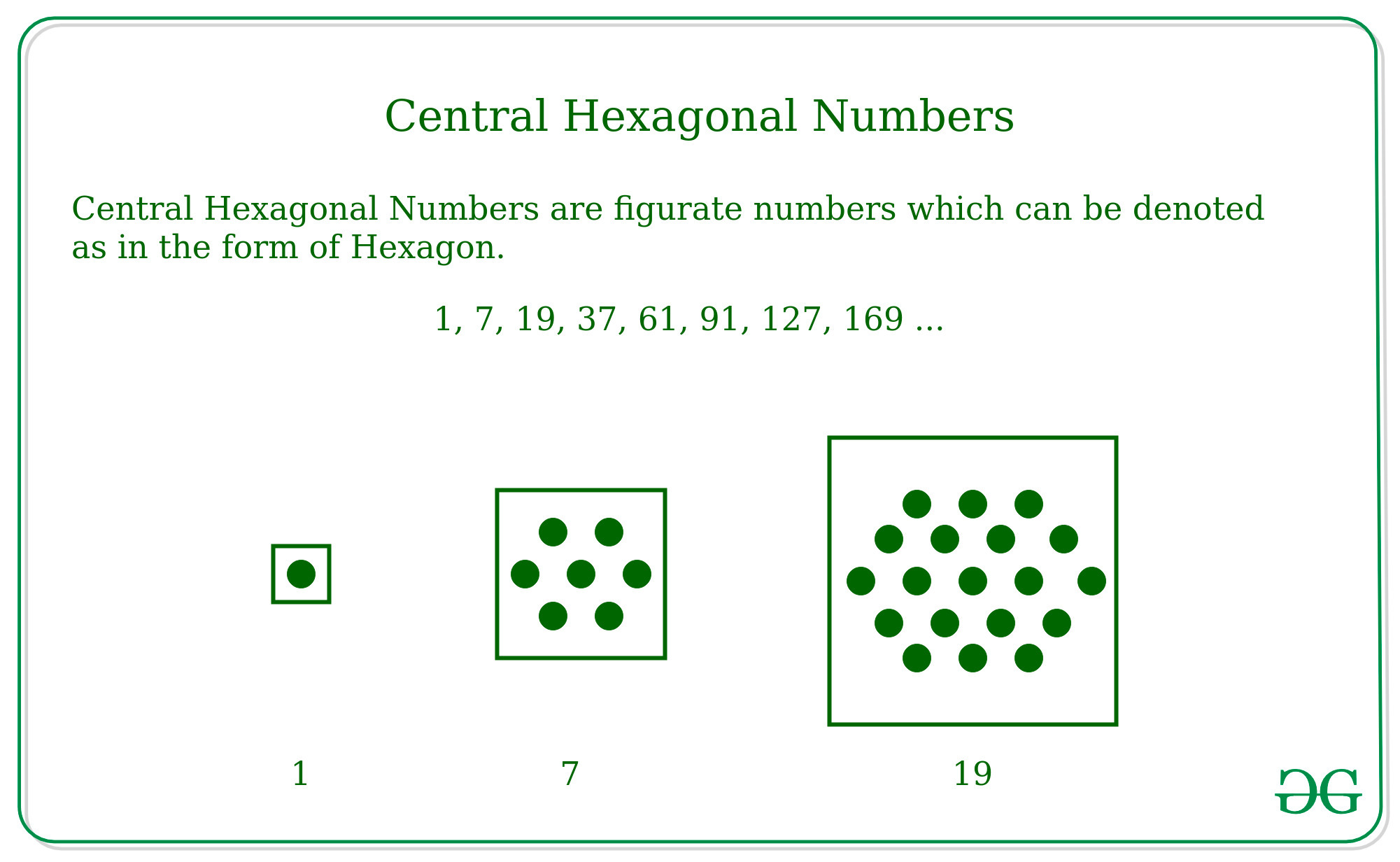Skip to content
Related Articles
Centered hexagonal number
• Last Updated : 08 Apr, 2021

Given a number N and the task is to find Nth centered hexagonal number. Also, find the Centered hexagonal series.
Examples:

Input: N = 2
Output: 7
Input: N = 10
Output: 271

Centered Hexagonal Numbers – The Centered Hexagonal numbers are figurate numbers and are in the form of the Hexagon. The Centered Hexagonal number is different from Hexagonal Number because it contains one element at the center.
Some of the Central Hexagonal numbers are –

`1, 7, 19, 37, 61, 91, 127, 169 ... `For Example:

```The First N numbers are -
1, 7, 19, 37, 61, 91, 127 ...

The cumulative sum of these numbers are -
1, 1+7, 1+7+19, 1+7+19+37...

which is nothing but the sequence -
1, 8, 27, 64, 125, 216 ...

That is in the form of  -
13, 23, 33, 43, 53, 63 ....```

As Central Hexagonal numbers sum up to Nth term will be the N3. That is –

13 + 23 + 33 + 43 + 53 + 63 …. upto N terms = N3
Then, Nth term will be –
=> N3 – (N – 1)3
=> 3*N*(N – 1) + 1

Approach: For finding the Nth term of the Centered Hexagonal Number use the formulae – 3*N*(N – 1) + 1.
Below is the implementation of the above approach:

## C++

 `// Program to find nth``// centered hexadecimal number.``#include ``using` `namespace` `std;` `// Function to find centered``// hexadecimal number.``int` `centeredHexagonalNumber(``int` `n)``{``    ``// Formula to calculate nth``    ``// centered hexadecimal number``    ``// and return it into main function.``    ``return` `3 * n * (n - 1) + 1;``}` `// Driver Code``int` `main()``{``    ``int` `n = 10;``    ``cout << n << ``"th centered hexagonal number: "``;``    ``cout << centeredHexagonalNumber(n);``    ``return` `0;``}`

## Java

 `// Java Program to find nth``// centered hexadecimal number``import` `java.io.*;` `class` `GFG``{``    ` `    ``// Function to find centered``    ``// hexadecimal number``    ``static` `int` `centeredHexagonalNumber(``int` `n)``    ``{``        ``// Formula to calculate nth``        ``// centered hexadecimal number``        ``// and return it into main function``        ``return` `3` `* n * (n - ``1``) + ``1``;``    ``}``    ` `    ``// Driver Code``    ``public` `static` `void` `main(String args[])``    ``{``        ``int` `n = ``10``;``        ``System.out.print(n + ``"th centered "` `+``                       ``"hexagonal number: "``);``        ``System.out.println(centeredHexagonalNumber(n));``        ` `    ``}``}` `// This code is contributed by Nikita Tiwari.`

## Python3

 `# Python 3 program to find nth``# centered hexagonal number` `# Function to find``# centered hexagonal number``def` `centeredHexagonalNumber(n) :``    ` `    ``# Formula to calculate``    ``# nth centered hexagonal``    ``return` `3` `*` `n ``*` `(n ``-` `1``) ``+` `1`  `# Driver Code``if` `__name__ ``=``=` `'__main__'` `:``        ` `    ``n ``=` `10``    ``print``(n, ``"th centered hexagonal number: "``                ``, centeredHexagonalNumber(n))`  `# This code is contributed``# by 'Akanshgupta'`

## C#

 `// C# Program to find nth``// centered hexadecimal number``using` `System;` `class` `GFG``{``    ` `    ``// Function to find centered``    ``// hexadecimal number``    ``static` `int` `centeredHexagonalNumber(``int` `n)``    ``{``        ``// Formula to calculate nth``        ``// centered hexadecimal number``        ``// and return it into main function``        ``return` `3 * n * (n - 1) + 1;``    ``}``    ` `    ``// Driver Code``    ``public` `static` `void` `Main()``    ``{``        ``int` `n = 10;``        ``Console.Write(n + ``"th centered "``+``                   ``"hexagonal number: "``);``        ``Console.Write(centeredHexagonalNumber(n));``        ` `    ``}``}` `// This code is contributed by vt_m.`

## PHP

 ``

## Javascript

 ``

Output :

`10th centered hexagonal number: 271`

Performance Analysis:

• Time Complexity: In the above given approach we are finding the Nth term of the Centered Hexagonal Number which takes constant time. Therefore, the complexity will be O(1)
• Space Complexity: In the above given approach, we are not using any other auxiliary space for the computation. Therefore, the space complexity will be O(1).

### Centered Hexagonal series

Given a number N, the task is to find centered hexagonal series till N.
Approach:
Iterate the loop using a loop variable (say i) and find the each ith term of the Centered Hexagonal Number using the formulae – 3*i*(i – 1) + 1
Below is the implementation of the above approach:

## C++

 `// Program to find the series``// of centered hexadecimal number``#include ``using` `namespace` `std;` `// Function to find the``// series of centered``// hexadecimal number.``void` `centeredHexagonalSeries(``int` `n)``{``    ``// Formula to calculate``    ``// nth centered hexadecimal``    ``// number.``    ``for` `(``int` `i = 1; i <= n; i++)``        ``cout << 3 * i * (i - 1) + 1``             ``<< ``" "``;``}` `// Driver Code``int` `main()``{``    ``int` `n = 10;``    ``centeredHexagonalSeries(n);``    ``return` `0;``}`

## Java

 `// Program to find the series of``// centered hexadecimal number.``import` `java.io.*;` `class` `GFG``{``    ``// Function to find the series of``    ``// centered hexadecimal number.``    ``static` `void` `centeredHexagonalSeries(``int` `n)``    ``{``        ``// Formula to calculate nth``        ``// centered hexadecimal number.``        ``for` `(``int` `i = ``1``; i <= n; i++)``            ``System.out.print( ``3` `* i *``                            ``(i - ``1``) + ``1` `+ ``" "``);``    ``}``    ` `    ``// Driver Code``    ``public` `static` `void` `main(String args[])``    ``{``        ``int` `n = ``10``;``        ``centeredHexagonalSeries(n);``    ``}``}` `// This code is contributed by Nikita Tiwari.`

## Python3

 `# Python3 program to find``# nth centered hexagonal number` `# Function to find centered hexagonal``# series till n given numbers.``def` `centeredHexagonalSeries(n) :``    ``for` `i ``in` `range``(``1``, n ``+` `1``) :``        ` `        ``# Formula to calculate nth``        ``# centered hexagonal series.``        ``print``(``3` `*` `i ``*` `(i ``-` `1``) ``+` `1``, end``=``" "``)``        ` `# Driver Code``if` `__name__ ``=``=` `'__main__'` `:``    ` `    ``n ``=` `10``    ``centeredHexagonalSeries(n)` `# This code is contributed``# by 'Akanshgupta'`

## C#

 `// C# Program to find the``// series of centered``// hexadecimal number.``using` `System;` `class` `GFG``{``    ` `    ``// Function to find the``    ``// series of centered``    ``// hexadecimal number.``    ``static` `void` `centeredHexagonalSeries(``int` `n)``    ``{``        ``// Formula to calculate nth``        ``// centered hexadecimal number.``        ``for` `(``int` `i = 1; i <= n; i++)``            ``Console.Write( 3 * i *``                         ``(i - 1) + 1 + ``" "``);``    ``}``    ` `    ``// Driver Code``    ``public` `static` `void` `Main()``    ``{``        ``int` `n = 10;``        ``centeredHexagonalSeries(n);``    ``}``}` `// This code is contributed by vt_m.`

## PHP

 ``

## Javascript

 ``

Output :

`1 7 19 37 61 91 127 169 217 271`

Attention reader! Don’t stop learning now. Get hold of all the important mathematical concepts for competitive programming with the Essential Maths for CP Course at a student-friendly price. To complete your preparation from learning a language to DS Algo and many more,  please refer Complete Interview Preparation Course.

My Personal Notes arrow_drop_up